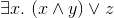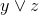# Verification of Software and Hardware using Quantified Boolean Formulas (QBF)

## Will Klieber

### Ph.D. Thesis

May 2014

Full Document: thesis.pdf

Abstract.

Many problems in formal verification of digital hardware circuits and other finite-state systems are naturally expressed in the language of quantified boolean formulas (QBF). This thesis presents advancements in techniques for QBF solvers that enable verification of larger and more complex systems.

Most of the existing work on QBF solvers has required that formulas be converted into prenex conjunctive normal form (CNF). However, the Tseitin transformation (which is used to convert a formula to CNF) is asymmetric between the two quantifier types. In particular, it introduces new existentially quantified variables, but not universally quantified variables. It turns out that this makes it harder for QBF solvers to detect when a formula has become true (but not when a formula has become false) under an assignment.

We present a technique using ghost variables that handles non-CNF QBF formulas using a symmetric alternative to the Tseitin transformation. We introduce sequent learning, a reformulation and generalization of clause/cube learning. With sequent learning, we can handle non-prenex formulas. Whereas clause/cube learning only allows learning when the whole input formula becomes true or false, sequent learning allows us to learn when a quantified subformula becomes true or false.

Almost all QBF research so far has focused on closed formulas, i.e., formulas without any free variables. Closed QBF formulas evaluate to either `true` or `false`. Sequent learning lets us extend existing QBF techniques to handle open formulas, which contain free variables. A solution to an open QBF formula is a quantifier-free formula that is logically equivalent to the original formula. For example, a solution to the open QBF formulais the formula.

The final part of this thesis discusses an approach to QBF that uses Counterexample-Guided Abstraction Refinement (CEGAR) to partially expand quantifier blocks. The approach recursively solves QBF instances with multiple quantifier alternations. Experimental results show that the recursive CEGAR-based approach outperforms existing types of solvers on many publicly-available benchmark families. In addition, we present a method of combining the CEGAR technique with a DPLL-based solver and show that it improves the DPLL solver on many benchmarks.